### 北京奔驰 奔驰GLC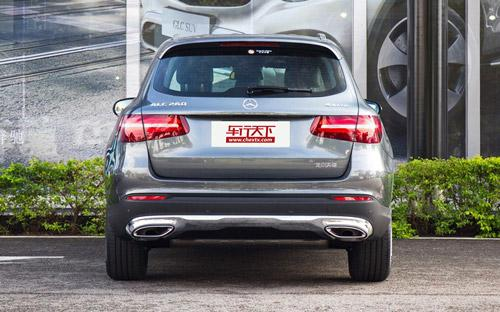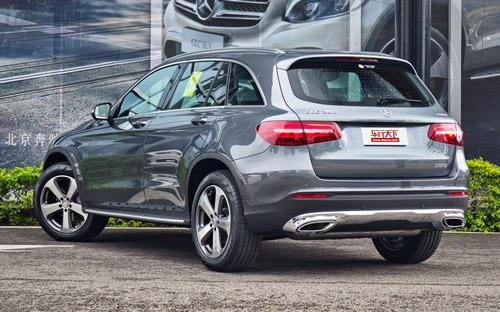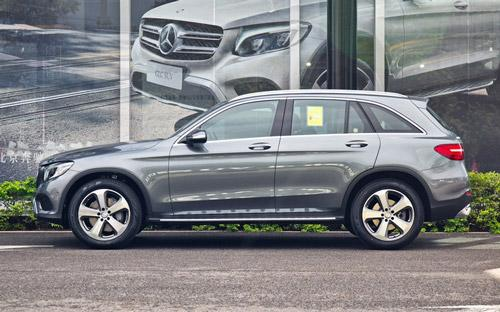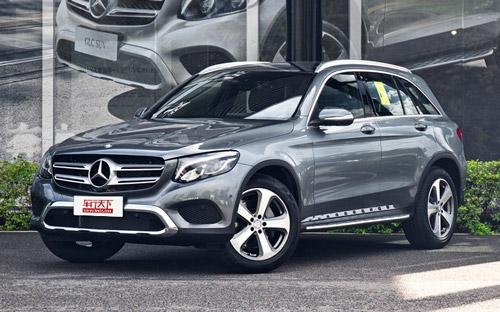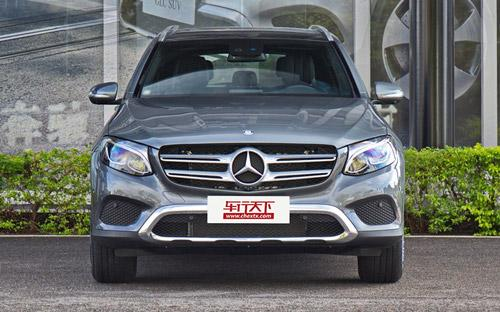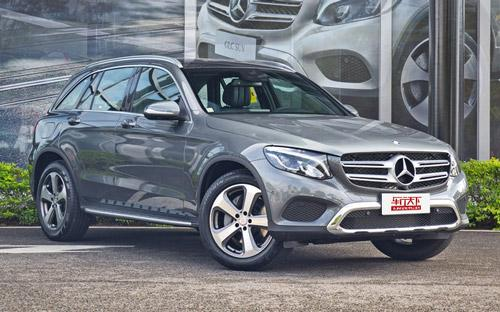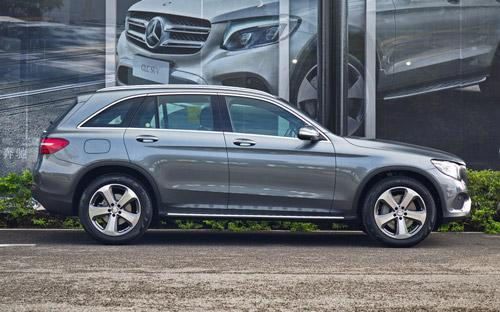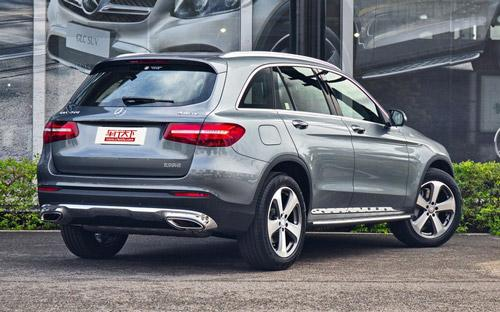8 种颜色可选2016款最低售价：39.60 万元起

4661(mm)1898(mm)1664(mm)##### 配置亮点：
• 胎压监测装置

• ISOFIX儿童座椅接口

• 车身稳定控制(ESC/ESP/DSC等)

• 电动天窗

• 定速巡航

• 后倒车雷达

• 真皮座椅

• GPS导航系统

• 氙气大灯

• 后视镜加热

• 提交
2016款 GLC 260 4MATIC 动感型 (155张)
• 2016款 GLC 260 4MATIC 动感型 (155张)
• 2016款 GLC 260 4MATIC 豪华型 (631张)
• 2016款 GLC 300 4MATIC 豪华型 (233张)
• 2016款 GLC 200 4MATIC (276张)
• 北京奔驰 奔驰GLC 绕车实拍• 北京奔驰 奔驰GLC 在售车型

排量 车型 厂商指导价 本地最低报价 购车工具
2.0L
GLC 200 4MATIC 9挡手自一体
39.60万
39.60万

GLC 300 4MATIC 豪华型 9挡手自一体
57.90万
57.90万

2.0T
GLC 260 4MATIC 动感型 9挡手自一体
42.80万
42.80万

GLC 260 4MATIC 豪华型 9挡手自一体
44.30万
GLC 300 4MATIC 动感型 9挡手自一体
48.60万

北京奔驰 奔驰GLC 经销商

查看更多 >>

### 北京奔驰 奔驰GLC 动力加速

奔驰GLC 0-100公里加速时间分布在 6.9-8.2秒 属于 高性能级

动力级别 加速时间 车型
运动级(2款)
8.2s
GLC 200 4MATIC
7.8s
GLC 260 4MATIC 动感型GLC 260 4MATIC 豪华型
高性能级(1款)
6.9s
GLC 300 4MATIC 豪华型GLC 300 4MATIC 动感型

北京奔驰 奔驰GLC 视频

北京奔驰 奔驰GLC 新闻资讯

# SUV持续增温 2016成都车展展台与重点车型前瞻

新闻 超过9184次关注

今年的第十九届展会将于9月2日至11日在成都世纪城新国际会展中心举行。本次车展的主题是“缤纷车展·炫动蓉城”，但相信没有太多人关注这个，因为大家的目光早已被...

# 首推260 4MATIC 动感型 奔驰GLC购车手册

汽车导购 超过10511次关注

与对手Q5与X3对比，全新换代的国产奔驰GLC无论是在整体技术先进性还是售价方面都很有竞争力，上市不久便成为该级别关注度最高的车型，那它在配置方面的表现又怎么样...

# 3种动力5款车型 国产GLC级部分配置曝光

新闻 超过9153次关注

我们获得了奔驰国产GLC级的部分配置，新车全系标配9速自动变速箱以及4MATIC四驱系统；发动机方面，新车仅搭载2.0T发动机，并提供三种不同动力调校共计5款车型供消费...

# 北京奔驰GLC上市 售价39.60-57.90万元

上市新车 超过9276次关注

北京奔驰GLC广州正式上市，国产GLC级延续了海外版车型的设计，使用了最新的家族式设计，并与新C级风格看齐，前大灯造型设计饱满，内饰设计豪华感提升，全系搭载M274...

# 搭载2.0T发动机 北京奔驰GLC级实车曝光

国产新车 超过7771次关注

我们从官方获得了一组北京奔驰国产GLC级的谍照，新车整体造型与海外版保持一致，国产奔驰GLC级的外观设计与海外版车型保持一致，前脸采用奔驰最新家族式风格，搭载...

猜你喜欢

﻿
• 快速找车
• 选择品牌
• 选择品牌
• A  奥迪
• A  阿斯顿·马丁
• A  阿尔法·罗密欧
• B  宝沃
• B  布加迪
• B  巴博斯
• B  保时捷
• B  宾利
• B  奔驰
• B  宝马
• B  本田
• B  别克
• B  标致
• B  比亚迪
• B  宝骏
• B  北汽制造
• B  北汽新能源
• B  北汽幻速
• B  北汽威旺
• B  北京汽车
• B  奔腾
• B  北汽绅宝
• C  长安
• C  长安商用
• C  长城
• C  昌河
• D  大众
• D  道奇
• D  DS
• D  东南
• D  东风风神
• D  东风风行
• D  东风小康
• D  东风风度
• D  东风
• F  福特
• F  丰田
• F  菲亚特
• F  法拉利
• F  福田
• F  福迪
• F  福汽启腾
• G  观致
• G  广汽传祺
• G  广汽吉奥
• G  GMC
• H  红旗
• H  汉腾汽车
• H  哈弗
• H  哈飞
• H  海格
• H  海马
• H  华颂
• H  黄海
• H  华泰
• H  恒天
• J  吉利汽车
• J  捷豹
• J  Jeep
• J  江淮
• J  江铃
• J  金杯
• J  九龙
• J  金旅
• K  凯翼
• K  凯迪拉克
• K  克莱斯勒
• K  科尼塞克
• K  卡威
• K  开瑞
• L  路虎
• L  林肯
• L  劳斯莱斯
• L  兰博基尼
• L  雷克萨斯
• L  铃木
• L  雷诺
• L  理念
• L  力帆
• L  莲花汽车
• L  猎豹
• L  路特斯
• L  陆风
• M  马自达
• M  MG
• M  MINI
• M  玛莎拉蒂
• M  摩根
• M  迈凯轮
• N  纳智捷
• O  欧宝
• O  讴歌
• O  欧朗
• Q  奇瑞
• Q  起亚
• Q  启辰
• R  日产
• R  荣威
• R  瑞麒
• S  三菱
• S  斯威汽车
• S  萨博
• S  smart
• S  斯柯达
• S  斯巴鲁
• S  思铭
• S  双龙
• S  上汽大通
• S  双环
• T  特斯拉
• T  腾势
• W  沃尔沃
• W  五菱汽车
• W  五十铃
• W  威兹曼
• W  威麟
• X  现代
• X  雪佛兰
• X  雪铁龙
• X  西雅特
• Y  一汽
• Y  英菲尼迪
• Y  英致
• Y  依维柯
• Y  野马汽车
• Y  永源
• Z  众泰
• Z  中华
• Z  中兴
• Z  知豆
• 选择车系
• 选择车系
• 车型对比
• 选择品牌
• 选择品牌
• A  奥迪
• A  阿斯顿·马丁
• A  阿尔法·罗密欧
• B  宝沃
• B  布加迪
• B  巴博斯
• B  保时捷
• B  宾利
• B  奔驰
• B  宝马
• B  本田
• B  别克
• B  标致
• B  比亚迪
• B  宝骏
• B  北汽制造
• B  北汽新能源
• B  北汽幻速
• B  北汽威旺
• B  北京汽车
• B  奔腾
• B  北汽绅宝
• C  长安
• C  长安商用
• C  长城
• C  昌河
• D  大众
• D  道奇
• D  DS
• D  东南
• D  东风风神
• D  东风风行
• D  东风小康
• D  东风风度
• D  东风
• F  福特
• F  丰田
• F  菲亚特
• F  法拉利
• F  福田
• F  福迪
• F  福汽启腾
• G  观致
• G  广汽传祺
• G  广汽吉奥
• G  GMC
• H  红旗
• H  汉腾汽车
• H  哈弗
• H  哈飞
• H  海格
• H  海马
• H  华颂
• H  黄海
• H  华泰
• H  恒天
• J  吉利汽车
• J  捷豹
• J  Jeep
• J  江淮
• J  江铃
• J  金杯
• J  九龙
• J  金旅
• K  凯翼
• K  凯迪拉克
• K  克莱斯勒
• K  科尼塞克
• K  卡威
• K  开瑞
• L  路虎
• L  林肯
• L  劳斯莱斯
• L  兰博基尼
• L  雷克萨斯
• L  铃木
• L  雷诺
• L  理念
• L  力帆
• L  莲花汽车
• L  猎豹
• L  路特斯
• L  陆风
• M  马自达
• M  MG
• M  MINI
• M  玛莎拉蒂
• M  摩根
• M  迈凯轮
• N  纳智捷
• O  欧宝
• O  讴歌
• O  欧朗
• Q  奇瑞
• Q  起亚
• Q  启辰
• R  日产
• R  荣威
• R  瑞麒
• S  三菱
• S  斯威汽车
• S  萨博
• S  smart
• S  斯柯达
• S  斯巴鲁
• S  思铭
• S  双龙
• S  上汽大通
• S  双环
• T  特斯拉
• T  腾势
• W  沃尔沃
• W  五菱汽车
• W  五十铃
• W  威兹曼
• W  威麟
• X  现代
• X  雪佛兰
• X  雪铁龙
• X  西雅特
• Y  一汽
• Y  英菲尼迪
• Y  英致
• Y  依维柯
• Y  野马汽车
• Y  永源
• Z  众泰
• Z  中华
• Z  中兴
• Z  知豆
• 选择车系
• 选择车系
• 选择车型
• 选择车型
• 意见反馈